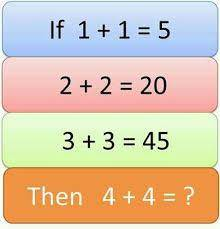### Can You Solve This? If 1+1 = 5, then 4 + 4 =?

Not as easy as it seems right? Drop your answer below showing how you arrived at it.#### Related Posts

###### After Paying N4.5million Annual Fees Per Student, Parents Reject N175,000 Monthly Fee Imposed for Online Learning by School

that group counting
1+1=5
2+2=20
3+3=45
4+4=80
Follow
2+2=10
3+3=15
4+4=20
6 months ago
• Abdullahi: 1+1=5
2+2=20
3+3=45
then 4+4 will now be 80.
4+4=80
5 months ago
80
Follow
1+1=5
2+2=20
3+3=45
4+4=80
Follow
1+1=5
2+2=20
3+3=45
4+4=80
1*1*5=5 AND 5 IS THE COMMON MULTIPLIER
2*2=4*5=20
3*3=9*5=45
4*4=16*5=80
Follow
1+1=5
1x1x5=5
2+2=20
2x2x5=20
3+3=45
3x3x5=45
4+4=?
4x4x5=80
Follow
70
Follow
1=2.5 if we use x to represent 1 so for second equation
4+4=y it will be 4(1)+4(1)= y so 4(2.5)+4(2.5)=y
y=20
Follow
1+1=5
2+2=20
3+3=45
4+4=80
Follow

#### Quick Questions

• 2 Answers   ·   4 hours ago
• 2 Answers   ·   4 hours ago
• 1 Answers   ·   3 hours ago

#### Post Comment

Please don't post or ask to join a "Group" or "Whatsapp Group" as a comment. It will be deleted. To join or start a group, please click here

1 Members
##### Marketplace
• Here are the things students around you have to sell;
• ###### Tecno t528
₦1,500   FCAA   Used